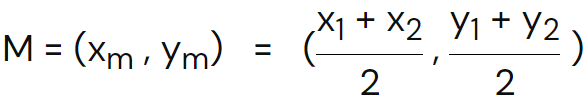# Midpoint Calculator

## How does midpoint calculator works?

To use the midpoint calculator, follow the steps below.

1. Enter the coordinate points of the line in the required input fields.
2. Hit the calculate button.
3. Press the reset button to enter new points.

Result

Data:

Formula:

Solution:

## Midpoint calculator

The midpoint calculator is used to calculate the middle point of a line segment with the help of the coordinate points of the line.

## What is the midpoint?

In mathematics, a point on a line segment that is cut into two congruent parts is known as the midpoint. It is the middle of the line segment. The midpoint is also defined as a point at or closer to the center of the line segment.

It bisects the line from the middle. It is related to coordinate geometry as it deals with the coordinate points of the line segment. The line segment is used to calculate the equation of the line by using the point-slope form or slope-intercept form.

### Formula of midpoint

The general expression of the midpoint is:Where

• Xm = is the midpoint of x-coordinates
• Ym = is the midpoint of y-coordinates

## How to calculate the midpoint?

Follow the below example to learn how to calculate the midpoint of the line segment.

Example

Find the midpoint of the line segment if the coordinate points of the line are (11, -12) & (-15, 14).

Step 1: First of all, write the coordinate points of x & y.

x1 = 11

y1 = -12

x2 = -15

y2 = 14

Step 2: Use the midpoint formula and calculate the midpoint of x & y coordinates.

Midpoint = (x1+x2)/2, (y1+y2)/2

For the midpoint of x-coordinate

(x1+x2)/2 = (11 + (-15))/2

(x1+x2)/2 = (11 – 15)/2

(x1+x2)/2 = -4/2 = -2

For the midpoint of the y-coordinate

(y1+y2)/2 = (-12 + 14)/2

(y1+y2)/2 = (2)/2

(y1+y2)/2 = 1

## Blogs9 months ago